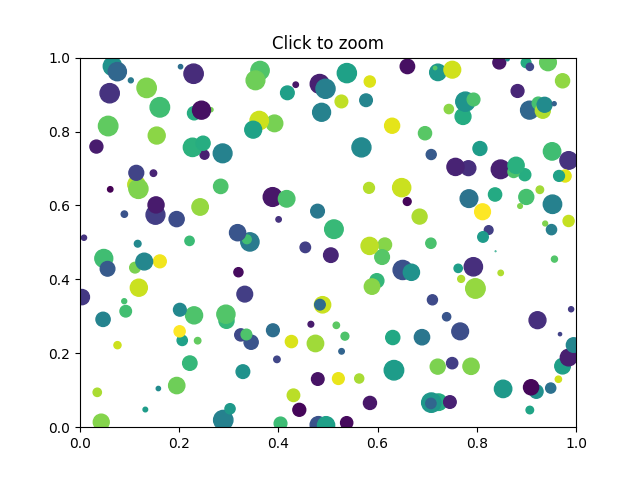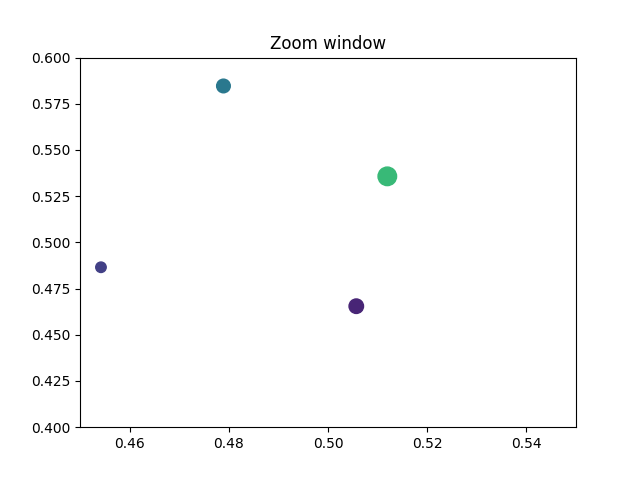Zoom Window¶

This example shows how to connect events in one window, for example, a mouse press, to another figure window.

If you click on a point in the first window, the z and y limits of the second will be adjusted so that the center of the zoom in the second window will be the x,y coordinates of the clicked point.

Note the diameter of the circles in the scatter are defined in points**2, so their size is independent of the zoom.

••import matplotlib.pyplot as plt
import numpy as np

figsrc, axsrc = plt.subplots()
figzoom, axzoom = plt.subplots()
axsrc.set(xlim=(0, 1), ylim=(0, 1), autoscale_on=False,
title='Click to zoom')
axzoom.set(xlim=(0.45, 0.55), ylim=(0.4, 0.6), autoscale_on=False,
title='Zoom window')

x, y, s, c = np.random.rand(4, 200)
s *= 200

axsrc.scatter(x, y, s, c)
axzoom.scatter(x, y, s, c)

def onpress(event):
if event.button != 1:
return
x, y = event.xdata, event.ydata
axzoom.set_xlim(x - 0.1, x + 0.1)
axzoom.set_ylim(y - 0.1, y + 0.1)
figzoom.canvas.draw()

figsrc.canvas.mpl_connect('button_press_event', onpress)
plt.show()

Keywords: matplotlib code example, codex, python plot, pyplot Gallery generated by Sphinx-Gallery